# Class 11 Maths NCERT Solutions for Chapter 12 Introduction to Three Dimensional Geometry Miscellaneous Exercise### Three Dimensional Geometry Miscellaneous Exercise Solutions

1. Three vertices of a parallelogram ABCD are A (3, –1, 2), B (1, 2, –4) and C (–1, 1, 2). Find the coordinates of the fourth vertex.

Solution

The three vertices of a parallelogram ABCD are given as A (3, –1, 2), B (1, 2, –4), and C (–1, 1, 2). Let the coordinates of the fourth vertex be D (x, y, z).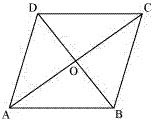We know that the diagonals of a parallelogram bisect each other.
Therefore, in parallelogram ABCD, AC and BD bisect each other.
∴ Mid - point of AC = Mid - point of BD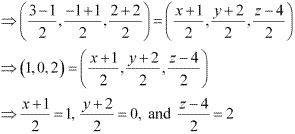⇒ x = 1, y = -2, and z = 8
Thus, the coordinates of the fourth vertex are (1, -2, 8).

2. Find the lengths of the medians of the triangle with vertices A (0, 0, 6), B (0, 4, 0) and (6, 0, 0).

Solution

Let AD, BE, and CF be the medians of the given triangle ABC.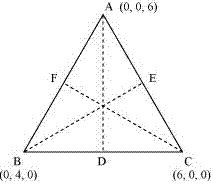Since AD is the median, D is the mid - point of BC.
∴ Coordinates of point D =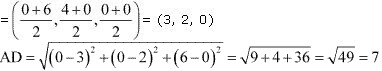Since BE is the median, E is the mid - point of AC.
∴ Coordinates of point E =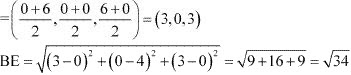Since CF is the median, F is the mid - point of AB.
∴ Coordinates of point F =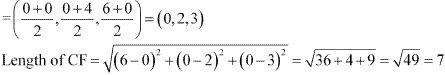3. If the origin is the centroid of the triangle PQR with vertices P (2a, 2, 6), Q (–4, 3b, –10) and R (8, 14, 2c), then find the values of a, b and c

Solution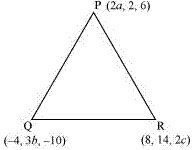It is known that the coordinates of the centroid of the triangle, whose vertices are (x1, y1, z1), (x2, y2, z2) and (x3, y3, z3), are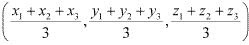Therefore, coordinates of the centroid of ΔPQR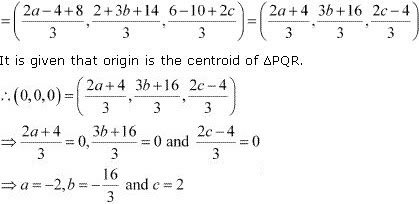Thus, the respective values of a, b and c are -2, -16/3, and  2.

4. Find the coordinates of a point on y-axis which are at a distance of 5√2 from the point P (3, –2, 5).
Solution

If a point is on the y-axis, then x-coordinate and the z-coordinate of the point are zero.
Let A (0, b, 0) be the point on the y-axis at a distance of 5√2 from point P (3, –2, 5).
Accordingly, AP = 5√2
∴ AP2 = 50
⇒ (3 -0)2 + (-2 - b)2 + (5 - 0)2 = 50
⇒ 9 + 4 + b2 + 4b + 25 = 50
⇒ b2 + 4b - 12 = 0
⇒ b2 + 6b - 2b - 12 = 0
⇒ (b + 6)(b - 2) = 0
⇒ b = -6 or  2
Thus, the coordinates of the required point are (0, 2, 0) and (0, -6, 0).

5. A point R with x-coordinate 4 lies on the line segment joining the pointsP (2, –3, 4) and Q (8, 0, 10). Find the coordinates of the point R.
[Hint suppose R divides PQ in the ratio k: 1. The coordinates of the point R are given by  [(8k + 2)/(k + 1) , -3/(k + 1) , (10k + 4)/(k + 1)]

Solution

The coordinates of points P and Q are given as P (2, –3, 4) and Q (8, 0, 10).
Let R divide line segment PQ in the ratio k:1.
Hence, by section formula, the coordinates of point R are given by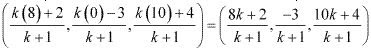It is given that the x - coordinate of point R is 4.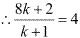⇒ 8k + 2 = 4k + 4
⇒ 4k = 2
⇒ k = 1/2
Therefore, the coordinates of point R are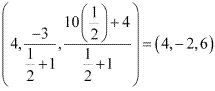6. If A and B be the points (3, 4, 5) and (–1, 3, –7), respectively, find the equation of the set of points P such that PA2 + PB2 = k2, where k is a constant.

Solution

The coordinates of points A and B are given as (3, 4, 5) and (–1, 3, –7) respectively.
Let the coordinates of point P be (x, y, z).
On using distance formula, we obtain
PA2 = (x - 3)2 + (y - 4)2 + (z - 5)2
= x2 + 9 - 6x + y2 + 16 - 8y + z2 + 25 - 10z
= x2 - 6x + y2 - 8y + z2 - 10z + 50
PB2 = (x + 1)2 + (y - 3)2 + (z + 7)2
= x2 + 2x + y2 - 6y + z2 + 14z + 59
Now, if PA2 + PB2 = k2 , then
(x2 - 6x + y2 - 8y + z2 - 10z + 50) + (x2 + 2x + y2 - 6y + z2 + 14z + 59) = k2
⇒ 2x2 + 2y2 + 2z2 - 4x - 14y + 4z + 109 = k2
⇒ 2(x2 + y2 + z2 - 2x - 7y + 2z) = k2 - 109
⇒ x2 + y2 + z2 - 2x - 7y + 2z = (k2 - 109)/2
Thus, the required equation is x2 + y2 + z2 - 2x - 7y + 2z = (k2 - 109)/2.